# Trees CS 5010 Program Design Paradigms Bootcamp Lesson

• Slides: 12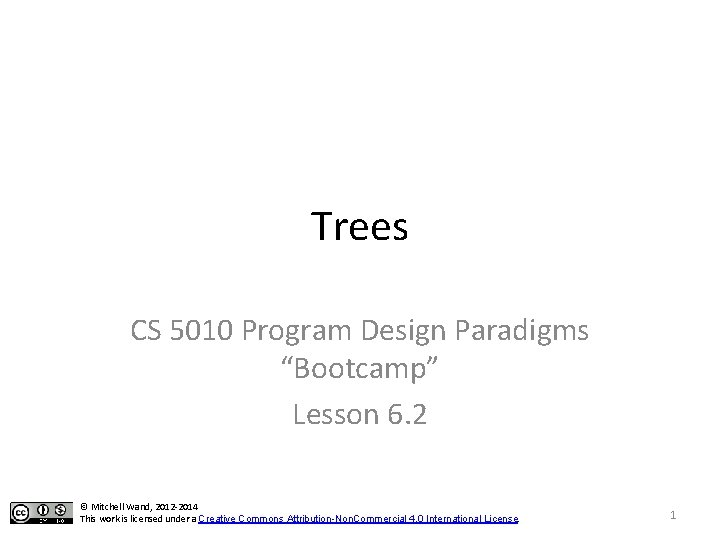Introduction/Outline • We've now learned about two ways to represent sequence information. • Many examples of information have a natural branching structure. • These are represented as trees, which you should have learned about in your data structures course. • In this lesson, we'll study how to apply the Design Recipe to trees. 2Learning Objectives • At the end of this lesson you should be able to: – Write a data definition for tree-structured information – Write a template for tree-structured information – Write functions that manipulate that data, using the template 3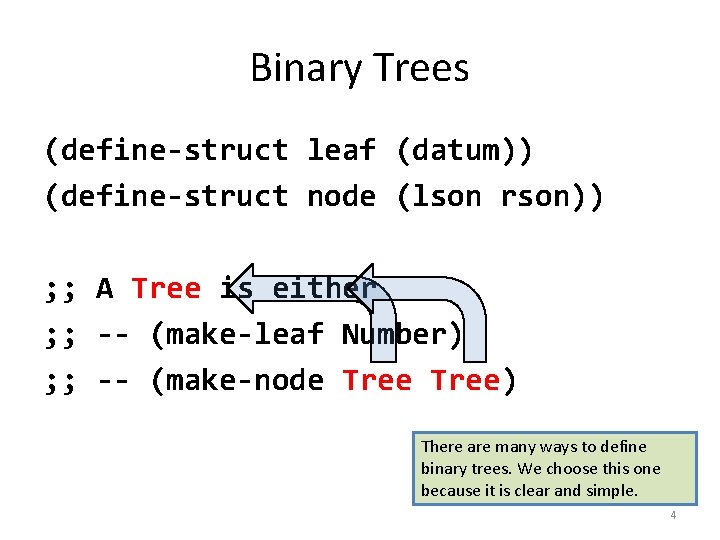Binary Trees (define-struct leaf (datum)) (define-struct node (lson rson)) ; ; A Tree is either ; ; -- (make-leaf Number) ; ; -- (make-node Tree) There are many ways to define binary trees. We choose this one because it is clear and simple. 4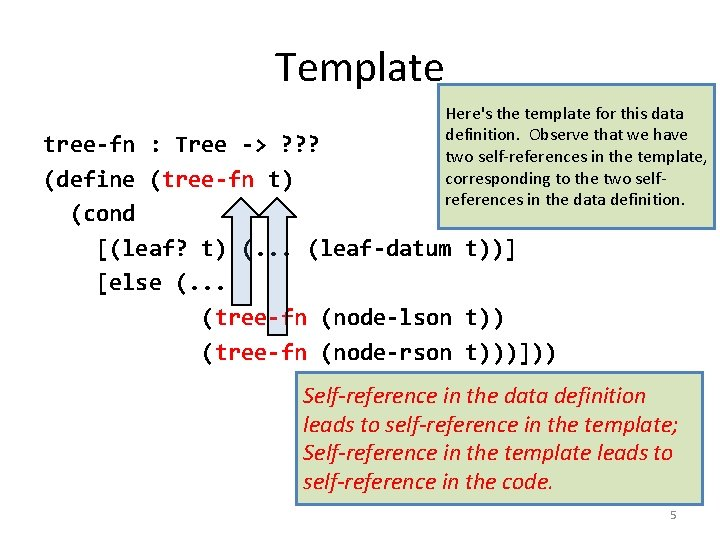Template Here's the template for this data definition. Observe that we have two self-references in the template, corresponding to the two selfreferences in the data definition. tree-fn : Tree -> ? ? ? (define (tree-fn t) (cond [(leaf? t) (. . . (leaf-datum t))] [else (. . . (tree-fn (node-lson t)) (tree-fn (node-rson t)))])) Self-reference in the data definition leads to self-reference in the template; Self-reference in the template leads to self-reference in the code. 5The template questions What’s the answer for a leaf? tree-fn : Tree -> ? ? ? (define (tree-fn t) (cond [(leaf? t) (. . . (leaf-datum t))] [else (. . . (tree-fn (node-lson t)) (tree-fn (node-rson t) ))])) If you knew the answers for the 2 sons, how could you find the answer for the whole tree? And here are the template questions. When we write a function using structural decomposition, we fill in the template with the answers to these questions. 6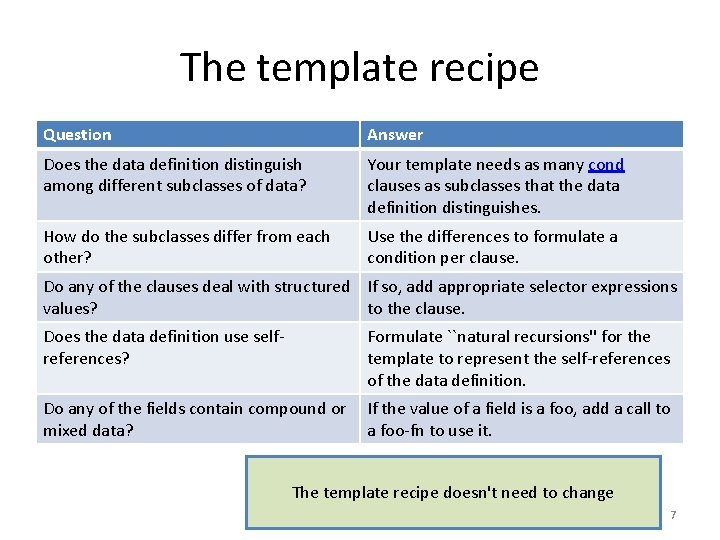The template recipe Question Answer Does the data definition distinguish among different subclasses of data? Your template needs as many cond clauses as subclasses that the data definition distinguishes. How do the subclasses differ from each other? Use the differences to formulate a condition per clause. Do any of the clauses deal with structured If so, add appropriate selector expressions values? to the clause. Does the data definition use selfreferences? Formulate ``natural recursions'' for the template to represent the self-references of the data definition. Do any of the fields contain compound or mixed data? If the value of a field is a foo, add a call to a foo-fn to use it. The template recipe doesn't need to change 7Next we'll do some examples of functions on binary trees. leaf-sum What’s the answer for a leaf? leaf-sum : Tree -> Number (define (leaf-sum t) (cond [(leaf? t) (leaf-datum t)] [else (+ (leaf-sum (node-lson t)) (leaf-sum (node-rson t)))])) If you knew the answers for the 2 sons, how could you find the answer for the whole tree? 8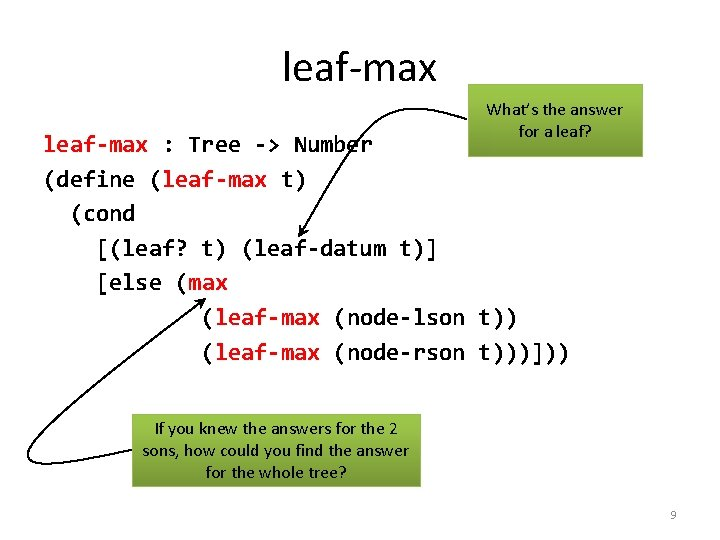leaf-max What’s the answer for a leaf? leaf-max : Tree -> Number (define (leaf-max t) (cond [(leaf? t) (leaf-datum t)] [else (max (leaf-max (node-lson t)) (leaf-max (node-rson t)))])) If you knew the answers for the 2 sons, how could you find the answer for the whole tree? 9leaf-min What’s the answer for a leaf? leaf-min : Tree -> Number (define (leaf-min t) (cond [(leaf? t) (leaf-datum t)] [else (min (leaf-min (node-lson t)) (leaf-min (node-rson t)))])) If you knew the answers for the 2 sons, how could you find the answer for the whole tree? 10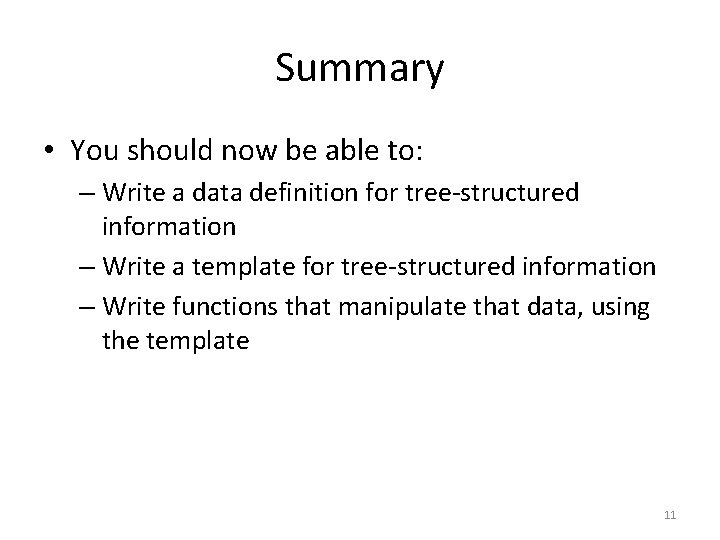Summary • You should now be able to: – Write a data definition for tree-structured information – Write a template for tree-structured information – Write functions that manipulate that data, using the template 11Next Steps • If you have questions about this lesson, ask them on the Discussion Board • Do Guided Practice 6. 2 • Go on to the next lesson 12